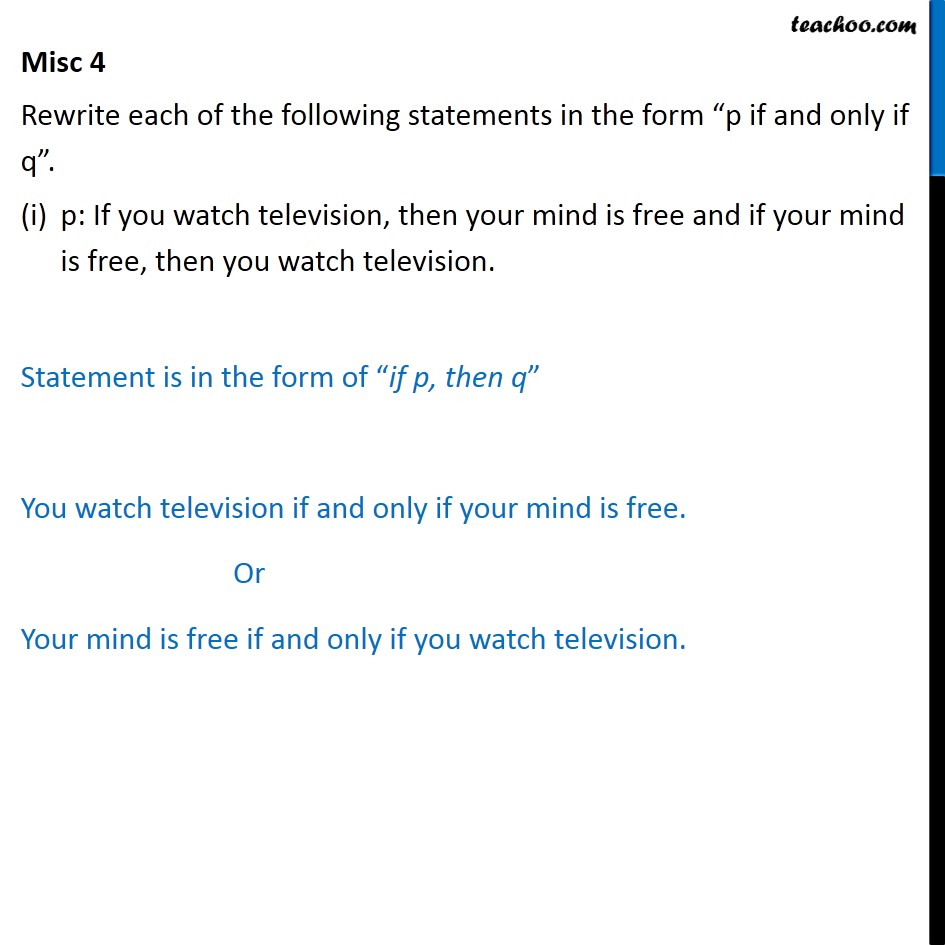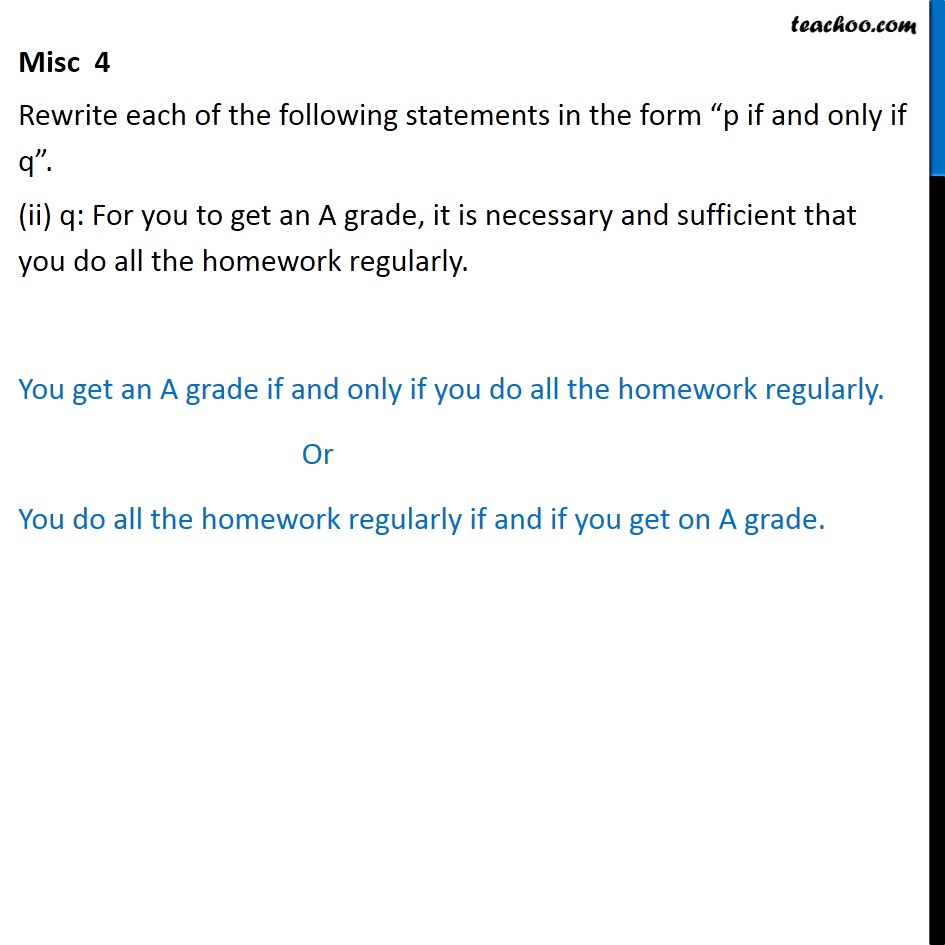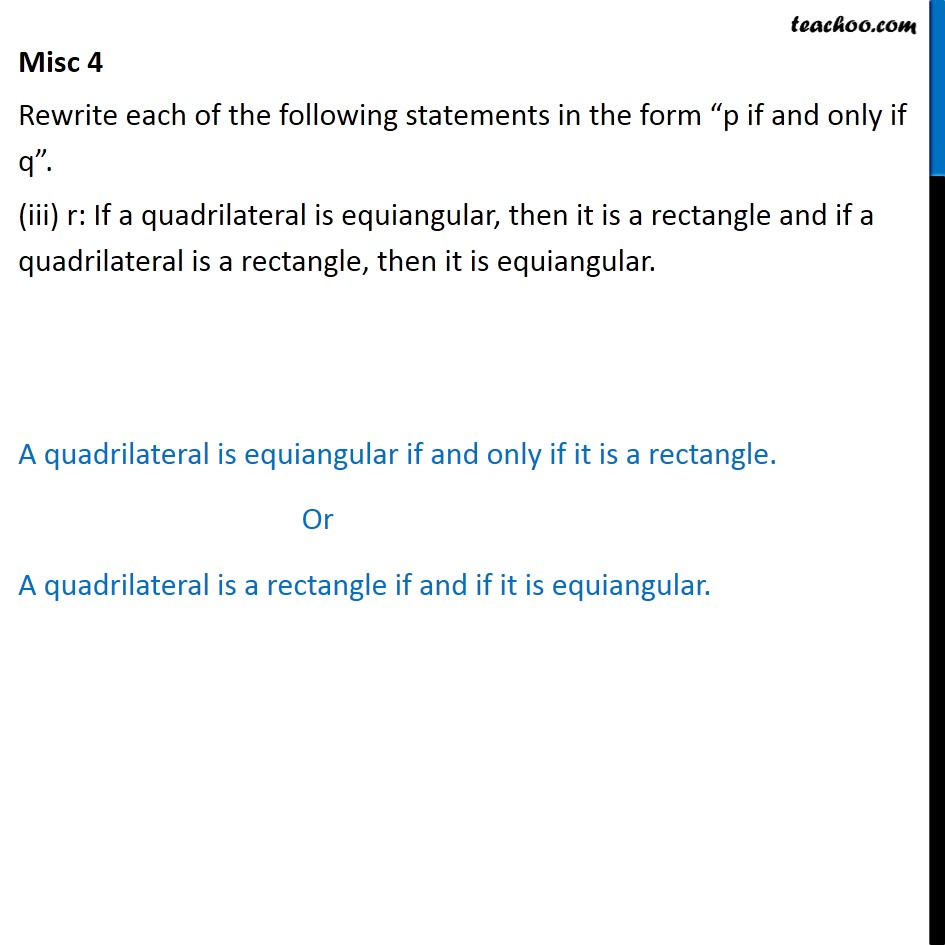1. Chapter 14 Class 11 Mathematical Reasoning
2. Serial order wise
3. Miscellaneous

Transcript

Misc 4 Rewrite each of the following statements in the form “p if and only if q”. p: If you watch television, then your mind is free and if your mind is free, then you watch television. Statement is in the form of “if p, then q” You watch television if and only if your mind is free. Or Your mind is free if and only if you watch television. Misc 4 Rewrite each of the following statements in the form “p if and only if q”. (ii) q: For you to get an A grade, it is necessary and sufficient that you do all the homework regularly. You get an A grade if and only if you do all the homework regularly. Or You do all the homework regularly if and if you get on A grade. Misc 4 Rewrite each of the following statements in the form “p if and only if q”. (iii) r: If a quadrilateral is equiangular, then it is a rectangle and if a quadrilateral is a rectangle, then it is equiangular. A quadrilateral is equiangular if and only if it is a rectangle. Or A quadrilateral is a rectangle if and if it is equiangular.

Miscellaneous

About the AuthorDavneet Singh
Davneet Singh is a graduate from Indian Institute of Technology, Kanpur. He has been teaching from the past 9 years. He provides courses for Maths and Science at Teachoo.